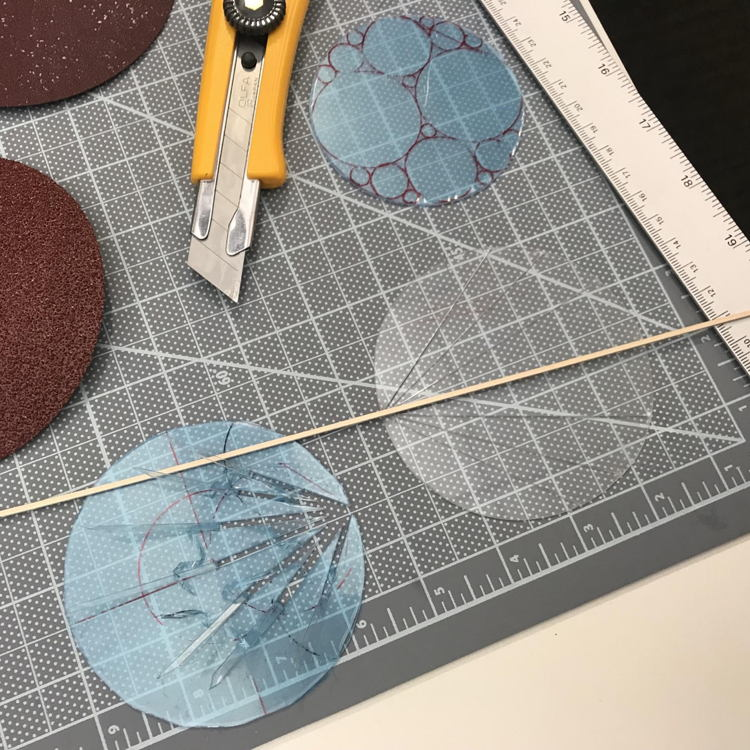# Communicating Infinities

Date started: 2019

###Abstract

Infinity is often misunderstood outside of the context of mathematics as something that is intuitively obvious ie. understood. By asking multiple mathematicians and non-mathematicians how they would describe infinity on paper, we can start comparing visualizations from different mathematical perspectives and non-mathematical interpretations to make an archive of useful symbolic visualizations for non-mathematicians and mathematicians of different uses and kinds of infinity.

### Media